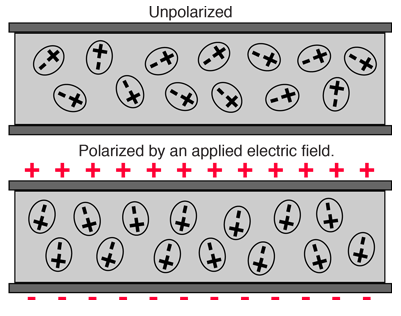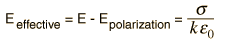# Polarization of Dielectric

If a material contains polar molecules, they will generally be in random orientations when no electric field is applied. An applied electric field will polarize the material by orienting the dipole moments of polar molecules.
 This decreases the effective electric field between the plates and will increase the capacitance of the parallel plate structure. The dielectric must be a good electric insulator so as to minimize any DC leakage current through a capacitor.The presence of the dielectric decreases the electric field produced by a given charge density.The factor k by which the effective field is decreased by the polarization of the dielectric is called the dielectric constant of the material.

 Effect on permittivity and capacitance.
Index

Capacitor Concepts

Dipole concepts

 HyperPhysics***** Electricity and Magnetism R Nave
Go Back

# Parallel Plate with Dielectric

The capacitance of a set of charged parallel plates is increased by the insertion of a dielectric material. The capacitance is inversely proportional to the electric field between the plates, and the presence of the dielectric reduces the effective electric field. The dielectric is characterized by a dielectric constant k, and the capacitance is multiplied by that factor.Calculation What is permittivity?
Index

Capacitor Concepts

 HyperPhysics***** Electricity and Magnetism R Nave
Go Back

# Parallel Plate with Dielectric

 When a dielectric is placed between charged plates, the polarization of the medium produces an electric field opposing the field of the charges on the plate. The dielectric constant k is defined to reflect the amount of reduction of effective electric field as shown below. The permittivity is a characteristic of space, and the relative permittivity or "dielectric constant" is a way to characterize the reduction in effective field because of the polarization of the dielectric. The capacitance of the parallel plate arrangement is increased by factor k.Index

Capacitor Concepts

 HyperPhysics***** Electricity and Magnetism R Nave
Go Back